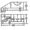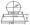# CSVTU Exam Papers – BE I Year – Engineering Graphics– Dec-Jan 2008-09

BE (2nd Semester)

Examination- Dec-Jan 2008-09

Engineering Graphics

UNIT- I

Q.1 (a) Define conic. What is eccentricity?

(b) A rectangular plot of land area 0.45 hectare is represented on a map by a similar rectangle of 5 sq. cm. Calculate the RF scale.

Also construct a scale to read up to a single meter & long enough to measure upto 400 mts: Mark the length of 257 m on

the  scale.

Or

A distance of 1 mm on a part of an instrument is represented by a line of 3 mm on drawing. Determine the RF of the

drawing construct a scale using the above RF, showing  tenth of mm, mm and cm and long enough to measure 5 cm. Mark on

it the distance of 35.7 mm.

(c) The foci of an ellipse are 90 mm apart and the minor axis is 65 mm long. Determine the length of major axis and draw the

ellipse by concentric circle method. Draw a tangent to the ellipse at a point on it 25 min above the major axis.

Or

One end of an inelastic thread of 120 mm length is attached to one; corner of a regular hexagonal disc having a side of 25mrr.

Draw the curve traced out by the other end of the thread when it is completely wound along the periphery of the disc, reeping

the thread always tight. Name the curve.

UNIT- II

Q.2 (a) How do you denote first angle projection method and third angle projection method using symbols?

(Solve any two):

(b) A line AB 90 mm long, is inclined at 30° to the H.P. Its end A is 12 mm above.the HP and 20 mm in front of V.P. Its fro it view

measure 65 mm. Draw the top view of AB, and determine its inclinations with the V.P. and also H.T, V.T.

(c) Three vertical poles AB, CD and EF are respectively 5,8 and 12 meter long their end B,D and F are on the ground and lie at the

comers of an equilateral triangle at 10 meters Ion ) side. Determine graphically the distance between the top ends if poles viz

AC, CE and EA.

(d) Two line AB and AC make an angle of 120° between them in this front view and top view. AB is parallel to both the HP and VP,

Determine the real angle between AB and AC.

UNIT- III

Q.3 (a) Show by means of traces, each of the following plane:

(i) Perpendicular to HP and inclined 30° to VP.

(ii)  Parallel to and 40 mm away from the VP.

(Solve any two) :

(b) Draw the projection of a regular pentagon of 40 mm side having its surface inclined at 30° to the H.P. and a side in the H.P. and

inclined at an angle of 60° to the V.P.

(c) A hexagonal pyramid base 25 side and axis 50 mm long has one of its triangular faces in the V.P. and the edge of the base

contained by that face makes an angle of 30° with the. H.P. Draw its projections.

(d) A cone diameter of base 50 mm and axis 50 mm long is resting on its base on the ground. It is cut by a section plane

perpendicular to the V.P. inclined at 75° to the HP and passing through the apex. Draw its front view, sectional top view

and true shape of the section.

UNIT- IV

Q.4 (a) A square pyramid, base 40 mm side and axis 65mm, long has its base on the ground with two edges parallel to the V.P. It is

cut by a section plane perpendicular to the V.P. and inclined 40° to the HP and bisecting the axis Draw development of the

surface of lower position of the pyramid.

(b)Draw the isometric view of the object shown in two views in following figuresOr

Draw the isometric of the paper weight with spherical knob shown in following figureUNIT- V

Q.5 (a) What is CADr ? Discuss its benefits.

(b) With the help of neat sketches , explain the use of the following autocad command :

(i) Rotate (ii) Offset (iii) Explode